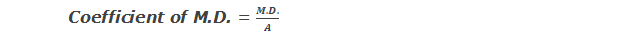# Mean Deviation

## Mean Deviation

Mean deviation or average deviation is defined as the arithmetic mean of the deviations of the items from mean or median or mode. Since median is the central point of any distribution, mean deviation from median generally gives the best result among the average deviations. Since mode is ill defined, mean deviation from mode will generally be not used.

********************

10 Math Problems officially announces the release of Quick Math Solver, an Android App on the Google Play Store for students around the world.

********************

### Individual Series:

In case of individual series, mean deviation is calculated as follows:
Where A = mean or median or mode and |x – A| is modulus or absolute value of x – A. That is magnitude of (x – A) or value of deviation taken from average A ignoring the negative sign.

### Discrete and Continuous Series:

In case of discrete and continuous series the mean deviation can be calculated by the formula,
In case of continuous distribution, x is taken as the mid-point of corresponding class.

### Coefficient of Mean Deviation

The coefficient of mean deviation is given by the formula,Where A is the average from which the mean deviation is calculated.

### Workout Examples

Example 1: Calculate the mean deviation and its coefficient from mean and median of the following series.
24, 28, 29, 33, 36, 35, 25

Solution: Here,
Arranging the data in ascending order,
Data: 24, 25, 28, 29, 33, 35, 36
No. of data (N) = 7
Calculation of Mean Deviation

 M.D. from mean M.D. from median x x – A |x – A| x x – A |x – A| 24 25 28 29 33 35 36 -6 -5 -2 -1 3 5 6 6 5 2 1 3 5 6 24 25 28 29 33 35 36 -5 -4 -1 0 4 6 7 5 4 1 0 4 6 7 ∑|x – A| = 28 ∑|x – A| = 27

Now,

Example 2: Calculate the mean deviation from median of the following frequency table. Also find its coefficient.

 Height (cm) 10 20 30 40 50 60 No. of plants 2 3 9 21 11 5

Solution: Here,
Calculation of Mean Deviation

 x f cf |x – A| f|x – A| 10 20 30 40 50 60 2 3 9 21 11 5 2 5 14 35 46 51 30 20 10 0 10 20 60 60 90 0 110 100 N = 51 420

Now,
No. of data (N) = 51
Hence, mean deviation from median is 8.235 and its coefficient is 0.205.

Example 3: Find the mean deviation from mean and its coefficient.

 Marks 10-20 20-30 30-40 40-50 50-60 60-70 70-80 No. of Students 4 7 9 18 12 7 3

Solution: Here,

 Marks Frequency (f) Mid-value (x) fx |x – A| f|x – A| 10-20 20-30 30-40 40-50 50-60 60-70 70-80 4 7 9 18 12 7 3 15 25 35 45 55 65 75 60 175 315 810 660 455 225 30 20 10 0 10 20 30 120 140 90 0 120 140 90 N = 60 2700 700

Here, total no. of data (N) = 60

Hence, mean deviation = 11.667 and its coefficient = 0.259.

You can comment your questions or problems regarding mean deviation here.

1.1.